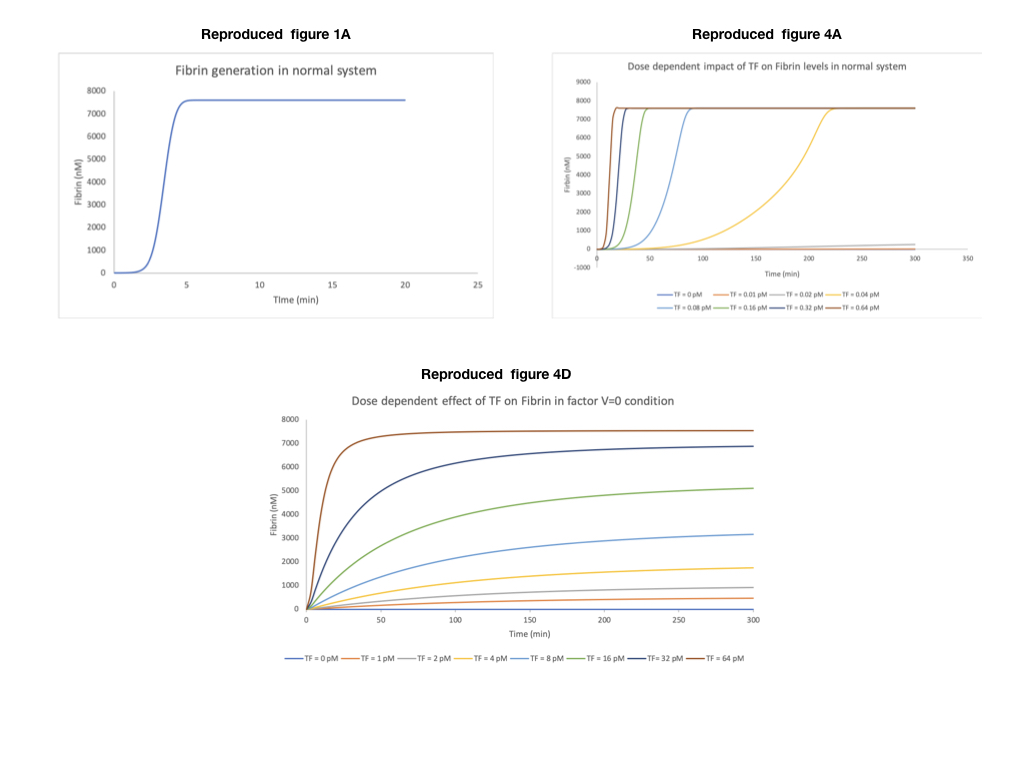## Panteleev2010 - Blood Coagulation: Full ModelModel Identifier
BIOMD0000000740
Short description
Full and reduced mathematical model of blood coagulation focusing on fibrin formation and the response to varied TF and V.
Format
SBML (L2V4)
Related Publication
• Task-oriented modular decomposition of biological networks: trigger mechanism in blood coagulation.• Panteleev MA, Balandina AN, Lipets EN, Ovanesov MV, Ataullakhanov FI
• Biophysical journal , 5/ 2010 , Volume 98 , Issue 9 , pages: 1751-1761 , PubMed ID: 20441738
• Center for Theoretical Problems of Physicochemical Pharmacology, Russian Academy of Sciences, Moscow, Russia. mapanteleev@yandex.ru
• Analysis of complex time-dependent biological networks is an important challenge in the current postgenomic era. We propose a middle-out approach for decomposition and analysis of complex time-dependent biological networks based on: 1), creation of a detailed mechanism-driven mathematical model of the network; 2), network response decomposition into several physiologically relevant subtasks; and 3), subsequent decomposition of the model, with the help of task-oriented necessity and sensitivity analysis into several modules that each control a single specific subtask, which is followed by further simplification employing temporal hierarchy reduction. The technique is tested and illustrated by studying blood coagulation. Five subtasks (threshold, triggering, control by blood flow velocity, spatial propagation, and localization), together with responsible modules, can be identified for the coagulation network. We show that the task of coagulation triggering is completely regulated by a two-step pathway containing a single positive feedback of factor V activation by thrombin. These theoretical predictions are experimentally confirmed by studies of fibrin generation in normal, factor V-, and factor VIII-deficient plasmas. The function of the factor V-dependent feedback is to minimize temporal and parametrical intervals of fibrin clot instability. We speculate that this pathway serves to lessen possibility of fibrin clot disruption by flow and subsequent thromboembolism.
Contributors
Submitter of the first revision: Matthew Roberts
Submitter of this revision: Krishna Kumar Tiwari
Modellers: Matthew Roberts, Krishna Kumar Tiwari

is (2 statements)
BioModels Database MODEL1808160002
BioModels Database BIOMD0000000740

isDescribedBy (1 statement)
PubMed 20441738

hasTaxon (1 statement)
Taxonomy Homo sapiens

isVersionOf (1 statement)
Gene Ontology blood coagulation

hasProperty (1 statement)
Mathematical Modelling Ontology Ordinary differential equation model

Curation status
Curated

Modelling approach(es)

Tags

#### Connected external resources

SBGN view in Newt Editor

Name Description Size Actions

### Model files

Panteleev2010_full_model.xml SBML L2V4 representation of Panteleev2010 - Blood Coagulation: Full Model 103.74 KB Preview | Download

• Model originally submitted by : Matthew Roberts
• Submitted: Aug 16, 2018 1:29:17 PM
##### Revisions

(*) You might be seeing discontinuous revisions as only public revisions are displayed here. Any private revisionsof this model will only be shown to the submitter and their collaborators.

Legends
: Variable used inside SBML models

Species
Species Initial Concentration/Amount
IXa

Coagulation Factor IX
0.0 nmol
V

Coagulation Factor V
0.0 nmol
II B

Thrombin
0.792569659442725 nmol
TF

Tissue Factor
0.005 nmol
Xa

Coagulation Factor X
0.0 nmol
TFPI

TFPI Gene
2.5 nmol
Xa VIIa TF

Coagulation Factor VII ; Coagulation Factor X ; Tissue Factor ; Coagulation Factor VII ; Coagulation Factor X
0.0 nmol
fibrinogen

Fibrinogen
7600.0 nmol
VII

Coagulation Factor VII
10.0 nmol
Va

Coagulation Factor V
0.0 nmol
Reactions
Reactions Rate Parameters
IXa = (k04/K04*IX*VIIa_TF_F+k05*IX*XIa/(K05+IX))-h03*Xa_TFPI*IXa (k04/K04*IX*VIIa_TF_F+k05*IX*XIa/(K05+IX))-h03*Xa_TFPI*IXa h03 = 8.2E-6; K05 = 200.0; k04 = 15.0; K04 = 210.0; k05 = 5.8
V = (-k16)*V*IIa_F/(K16+IIa_F) (-k16)*V*IIa_F/(K16+IIa_F) k16 = 14.0; K16 = 71.7
II_B = II*Phospholipid*n25/(K26*(1+X/K25+II/K26)) [] K26 = 470.0; n25 = 16000.0; K25 = 320.0
TF = (-(k01*VIIa*TF-k_01*VIIa_TF_F))-(k01*VII*TF-k_01*VII_TF) (-(k01*VIIa*TF-k_01*VIIa_TF_F))-(k01*VII*TF-k_01*VII_TF) k_01 = 1.1; k01 = 4.2
Xa = (((k06/K06*X*VIIa_TF_F+k07*IXa_B_F*X_B/(Phospholipid*K07)+k08*IXa_B_F*VIIIa_B_F*X_B/(Phospholipid^2*K09*k08))-(k11*Xa_F*TFPI-k_11*Xa_TFPI))-(h04*AT_III+h05*alpha2_macroglobulin+h06*alpha1_antitrypsin+h07*ProteinC_Inhibitor)*Xa_F)-h08*AT_III*Xa_Va_b (((k06/K06*X*VIIa_TF_F+k07*IXa_B_F*X_B/(Phospholipid*K07)+k08*IXa_B_F*VIIIa_B_F*X_B/(Phospholipid^2*K09*k08))-(k11*Xa_F*TFPI-k_11*Xa_TFPI))-(h04*AT_III+h05*alpha2_macroglobulin+h06*alpha1_antitrypsin+h07*ProteinC_Inhibitor)*Xa_F)-h08*AT_III*Xa_Va_b k11 = 0.052; h08 = 2.2E-5; h07 = 0.0012; k_11 = 0.02; K06 = 238.0; K07 = 230.0; h05 = 4.0E-5; k07 = 0.06; k08 = 6350.0; h04 = 1.5E-4; k06 = 435.0; h06 = 1.36E-5; K09 = 278.0
TFPI = (-(k11*Xa_F*TFPI-k_11*Xa_TFPI))-h02*Xa_VIIa_TF*TFPI (-(k11*Xa_F*TFPI-k_11*Xa_TFPI))-h02*Xa_VIIa_TF*TFPI k11 = 0.052; k_11 = 0.02; h02 = 6.0
Xa_VIIa_TF = k06/(K06*k_19)*X*VIIa_TF_F [] k06 = 435.0; k_19 = 770.0; K06 = 238.0
fibrinogen = (-k14/K14)*fibrinogen*IIa_F (-k14/K14)*fibrinogen*IIa_F k14 = 5040.0; K14 = 7200.0
VII = (-(k01*VII*TF-k_01*VII_TF))-k02*VII*IIa_F (-(k01*VII*TF-k_01*VII_TF))-k02*VII*IIa_F k_01 = 1.1; k02 = 0.0014; k01 = 4.2
Va = k16*V*IIa_F/(K16+IIa_F)-h15*APC*Va_B_F k16*V*IIa_F/(K16+IIa_F)-h15*APC*Va_B_F k16 = 14.0; K16 = 71.7; h15 = 7.7Curator's comment:
(added: 05 Jul 2019, 11:29:54, updated: 05 Jul 2019, 11:29:54)
figure 1a, figures 4A and figure 4 D reproduced front the literature as per the initial conditions provided by the literature. Model built and simulation done using COPASI 4.24 (build 197). Submitted .cps file can be directly to reproduce figure 1. For other figures, species concentration need to be modified as per given literature values.